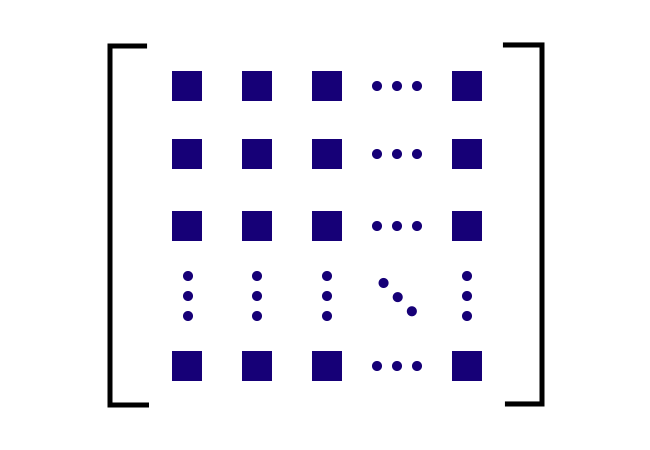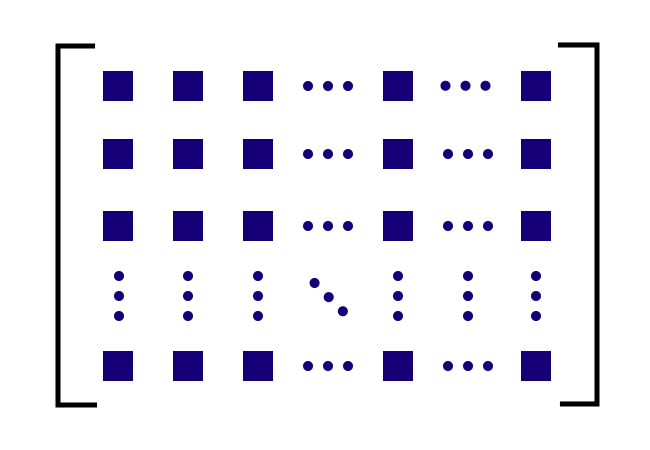# Main diagonal

A straight path that connects the elements (or entries) whose row and column are the same in a matrix is called the main diagonal of a matrix.

## Introduction

In a matrix, the elements can be arranged in rows and columns to express the entries in matrix form. In every row or column, there is an element (or entry) whose row and column are the same and those elements can be connected diagonally by a straight path and the diagonal straight path is called the main diagonal of the matrix.

Alternatively, the main diagonal of a matrix is also called by the following four names.

1. Principal diagonal
2. Primary diagonal
4. Major diagonal

In mathematics, the elements can be arranged possibly in rectangle or square form in a matrix. So, let’s learn how to identify the main diagonal in both types of matrices with understandable examples.

### Square matrix

For example, we have a square matrix of the order $3 \times 3$. The matrix contains $9$ elements and they are arranged in $3$ rows and $3$ columns.$\begin{bmatrix} \color{red} 5 & 2 & 9 \\ 8 & \color{red} 1 & 7 \\ 0 & 3 & \color{red} 4 \\ \end{bmatrix}$

However, there are three entries whose row and column are the same.

1. The element $5$ whose row is one and column is one.
2. The element $1$ whose row is two and column is two.
3. The entry $4$ whose row is three and column is column is three.

The three elements can be connected diagonally by a straight path and it is called a main diagonal of the square matrix.

### Rectangular matrix

For example, we have a rectangular matrix of the order $3 \times 4$. The matrix consists of $12$ elements, which are arranged in $3$ rows and $4$ columns.$\begin{bmatrix} \color{red} 3 & 1 & 8 & 2 \\ 7 & \color{red} 0 & 4 & 5 \\ 8 & 6 & \color{red} 5 & 1 \\ \end{bmatrix}$

However, there are three elements whose row and column are the same.

1. The entry $3$ whose row is one and column is one.
2. The element $0$ whose row is two and column is two.
3. The entry $5$ whose row is three and column is column is three.

The three elements can be connected by a straight path diagonally and the diagonal straight path is called the principal or leading diagonal of the rectangular matrix.

Latest Math Topics
Jun 26, 2023
Jun 23, 2023

###### Math Questions

The math problems with solutions to learn how to solve a problem.

Learn solutions

Practice now

###### Math Videos

The math videos tutorials with visual graphics to learn every concept.

Watch now

###### Subscribe us

Get the latest math updates from the Math Doubts by subscribing us.